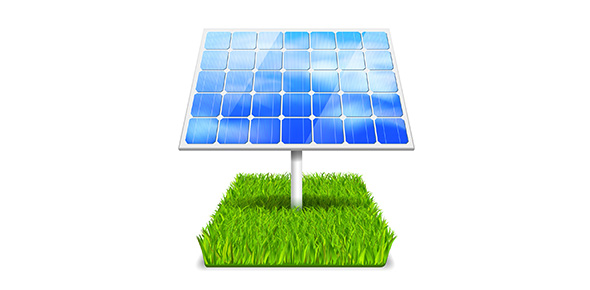# Mixed Science Quiz- Matter, Natural Cycles, And Energy

18 Questions | Total Attempts: 184SettingsCreate your own QuizA science quiz derived from our science book (GCF Reference book Gr. 6) This is a short review for we GCFers for our exams and hopefully for other users of ProProfs as well. Take your time and review your answers so that you may get high scores. Good luck!

• 1.
What are the two main things that power up the water cycle?
• A.

Gravity

• B.

Water

• C.

Sun

• 2.
How does Carbon return to the atmosphere?
• A.

Respiration of Plants

• B.

Respiration of animals

• C.

Decay

• D.

Fossil fuels being burned

• E.

Volcanic eruptions

• F.

Popping a balloon

• 3.
What is the sign of carbon dioxide?
• A.

Co

• B.

CO2

• C.

CH4

• 4.
The nitrogen in the atmosphere can be transformed into ________________ by nitrogen fixing bacteria.
• 5.
Define Mass and Volume in matter.
• A.

Mass- measure of amount of matter in an object Volume- the measure of the liquid inside it.

• B.

Mass- the amount of space something occupies Volume- The amount of matter inside it.

• C.

Mass- the quantitiy of matter inside it Volume- the space it occupies

• 6.
What are the three components of the Kinetic Molecular Theory of Matter?
• A.

Matter is composed of small particles.

• B.

A molecule is the smallest part of an atom.

• C.

A molecule is the smallest part of matter.

• D.

Each particle is always moving.

• E.

There is a space that separates the particles of matter

• 7.
Give examples of the Physical Property of Matter.
• A.

Color

• B.

Density

• C.

Odor

• D.

Freezing point

• E.

Flammability

• F.

Corrosiveness

• 8.
Solid to liquid is called fusion.
• A.

True

• B.

False

• 9.
The phase transition of Solid to Gas is called deposition.Giv
• A.

True

• B.

False

• 10.
Give at least one clue of chemical reaction/change.
• A.

Formation of bubbles

• B.

Freezing

• C.

Melting

• 11.
Energy is the capability to do ________.
• 12.
All electromagnetic radiations travel at an estimated speed of:
• A.

300000 meters per second

• B.

300000000 km/second

• C.

300000000 m/second

• 13.
What is potential and kinetic energy?
• A.

Potential- energy at rest Kinetic- energy ready for motion

• B.

Potential- Energy at rest Kinetic- Energy at motion

• C.

Potential- Energy at motion Kinetic- Energy at rest

• 14.
Sound energy is the energy when ____________________________________ in an organized way. ( 4 words)
• 15.
The Law of conservation of energy says that:
• A.

Energy can only be conserved not made nor destroyed

• B.

Energy can only be made and destroyed.

• C.

Energy can only be transformed, not made nor destroyed

• 16.
Examples of Non- renewable sources?
• A.

Biomass

• B.

Coal

• C.

Nuclear Plants

• D.

Geothermal

• E.

Hot spring

• F.

• 17.
Nuclear Energy comes mainly from:
• A.

Uraniun

• B.

Uranium

• C.

Coal

• D.

Fire

• 18.
Biomass is living and recently dead biological material that can be used as/for _________________________________. (1 or 2 words)
Related TopicsBack to top
×

Wait!
Here's an interesting quiz for you.